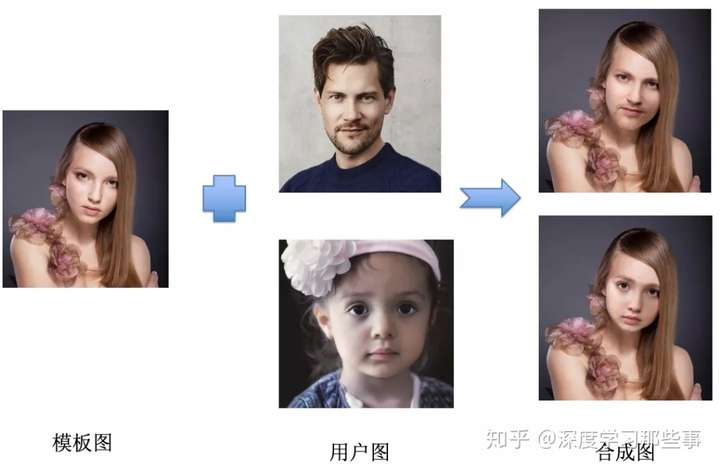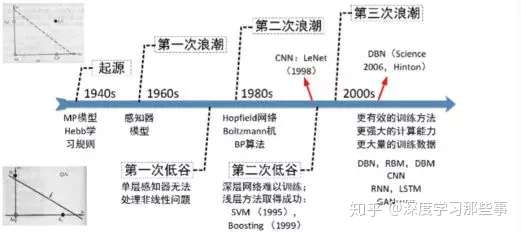# TensorFlow深度学习入门笔记（一）写在前面——如何入门深度学习

1.感性认识

2.了解概况

3.先跑起来

4.完成任务

5.技术细节

6.原理追溯TensorFlow2.0预览版已经公布了，有机会也会尝尝鲜import tensorflow as tf
import numpy as np
import matplotlib.pyplot as plt

# 添加神经网络层
Weights = tf.Variable(tf.random_normal([in_size,out_size]))
biases = tf.Variable(tf.zeros([1,out_size]) + 0.1)
Wx_plus_b = tf.matmul(inputs,Weights) + biases

if activation_function is None:
outputs = Wx_plus_b
else:
outputs = activation_function(Wx_plus_b)
return outputs

# 构建
x_data = np.linspace(-1,1,300)[:,np.newaxis]
noise = np.random.normal(0,0.05,x_data.shape)
y_data = np.square(x_data) - 0.5 + noise

xs = tf.placeholder(tf.float32,[None,1])
ys =tf.placeholder(tf.float32,[None,1])

# 建立输入层 隐藏层 输出层
loss = tf.reduce_mean(tf.reduce_sum(tf.square(ys - prediction),reduction_indices=))
# 预测
fig = plt.figure()
ax.scatter(x_data, y_data)
plt.ion()
plt.show()

sess = tf.Session()
sess.run(init)
for i in range(1000):
# training
sess.run(train_step, feed_dict={xs: x_data, ys: y_data})
if i % 20 == 0:
# to visualize the result and improvement
try:
ax.lines.remove(lines)
except Exception:
pass
prediction_value = sess.run(prediction, feed_dict={xs: x_data})
# plot the prediction
lines = ax.plot(x_data, prediction_value, 'r-', lw=5)
plt.pause(0.01)

print('over.')
sess.close()TensorFlow官网

https://www.jianshu.com/p/2a5936c2a6dd